# 38 Practice Exercises

## Multiple Choice Questions

Exercise: Endothermic Energy Balance

Consider a reactor in which an endothermic reaction takes place and 15.8 kJ are absorbed. A mixer is used in the reactor and provides 6.3 kJ of work on the system. What is the overall heat that needs to be provided to the system?

a) -22.1 kJ

b) 9.5 kJ

c) 22.1 kJ

d) -9.5 kJ

### Solution

b) 9.5 kJ
Performing an energy balance on the system, we know that $\Delta H = Q + W$. Since this is an endothermic reaction and work is done on the system, both the enthalpy of the reaction and the work are positive:
\begin{align*}
Q&= \Delta H -W \\
&= 15.8 kJ – 6.3 kJ\\
&=9.5kJ
\end{align*}

Exercise: Heat Capacity

A heat exchanger heats methanol from $25^{\circ}C$ to $100^{\circ}C$ at a pressure of 1 atm. The methanol is entering and exiting the heat exchanger at a molar flow rate of 500 mol/s. The following data is available:

 Cp (liquid) [J/molK] 80 Cp (vapour) [J/molK] 40 Heat of Vapourization [kJ/mol] 40 Normal Boiling Point Temperature $^{\circ}C$ 65

What is the rate of change in enthalpy for the heat exchange?

a) 22.3 MW

b) 2.3 MW

c) 2.3 kW

d) 22.3 kW

### Solution

a) 22.3 MW

Recall that $\Delta \dot{H} = \dot{n} \int_{T_{1}}^{T_{2}} CpdT$. We can expand this expression in order to include phase changes:

$\Delta \dot{H} = \dot{n} (\int_{T_{1}}^{T_{boiling}} Cp(liquid)dT + \Delta H_{vap} +\int_{T_{boiling}}^{T_{2}} Cp(vapour)dT )$

Plugging in our values, we get:

$\Delta \dot{H} = (500 mol/s) (\int_{25^{\circ}C}^{65^{\circ}C} 80 J/molK dT + 40,000 J/mol +\int_{65^{\circ}C}^{100^{\circ}C} 40 J/molK dT)$

$\Delta \dot{H} = (500 mol/s) (80 J/molK (65-25)K + 40,000 J/mol +40 J/molK (100-65)K )$

$\Delta \dot{H} = (500 mol/s)(44,600 J/mol)$

$\Delta \dot{H} = 22,300,000 J/s = 22,300,000 W = 22.3 MW$

Exercise: Utilities

We have the following utility options for heating toluene from $10^{\circ}C$ to $60^{\circ}C$.

 Utility Inlet T($^{\circ}C$) Outlet T($^{\circ}C$) Cost (\$/GJ) Cooling Water 20 25 0.378 Refrigerated Water 5 15 4.77 Low-pressure Steam 125 124 4.54 Medium-pressure Steam 175 174 4.77

Which utility would be the best option for this scenario?

a) Cooling water

b) Refrigerated water

c) Low-pressure steam

d) medium-pressure steam

### Solution

c) Low-pressure steam

A minimum of $10^{\circ}C$ of difference in temperature is required for efficient heat exchange.

The cooling and refrigerated water are not hot enough to heat the toluene. Both the low and medium pressure steam are hot enough to heat the toluene to $60^{\circ}C$, but low-pressure steam is the cheaper option.

Exercise: Hess’s Law

Consider the following desired reaction:

$3NO_{2} (g) + H_{2}O (l) → 2HNO_{3}(aq) + NO(g)$

Express the enthalpy change of the desired reaction using the following reactions’ enthalpies:

• Reaction 1: $2NO(g) + O_{2}(g) → 2NO_{2}(g)$ with $\Delta H_{1}$
• Reaction 2: $2N_{2}(g) + 5O_{2}(g) + 2H_{2}O(l) → 4HNO_{3}(aq)$ with $\Delta H_{2}$
• Reaction 3: $N_{2}(g) + O_{2}(g) → 2NO(g)$ with $\Delta H_{3}$

### Solution

Using Hess’s Law we can multiply reaction 1 by -3/2, reaction 2 by 1/2, and reaction 3 by -1 to obtain our desired reaction:

\begin{align*}
3NO_{2}(g) → 3NO(g) + \frac{3}{2}O_{2}(g) \;\;\;\;\;&-3/2\Delta H_{1}\\
N_{2}(g) + \frac{5}{2}O_{2}(g) + H_{2}O(l) → 2HNO_{3}(aq)\;\;\;\;\;&1/2\Delta H_{2}\\
2NO(g) → N_{2}(g) + O_{2}(g)\;\;\;\;\;&-\Delta H_{3}
\end{align*}

Therefore, our change in enthalpy can be represented by:

$\Delta H = -3/2*\Delta H_{1} + 1/2 \Delta H_{2} - \Delta H_{3}$

Exercise: Heat of Formation

Consider the combustion of acetylene. Find the enthalpy of combustion using the heats of formation at $25^{\circ} C$:

2C2H2(g) + 5O2(g) 4CO2(g) + 2H2O(g)

 Compound $\Delta H_{f}^{\circ}$ (kJ/mol) C2H2(g) 226.7 O2(g) 0 CO2(g) -393.5 H2O(g) -241.8

### Solution

Recall that

$\Delta H^{\circ}_{r} = \Sigma_{i}\nu_{i}\Delta\hat{H}^{\circ}_{f,i} = \Sigma_{products}|\nu_{i}|\Delta\hat{H}^{\circ}_{f,i}-\Sigma_{reactants}|\nu_{i}|\Delta\hat{H}^{\circ}_{f,i}$

Using the data provided and the reaction’s stoichiometry:

$\Delta H^{\circ}_{r} = 4 mol(-393.5 kJ/mol) + 2 mol(-241.8 kJ/mol) - 2 mol (226.7 kJ/mol) - 5 mol (0 kJ/mol)$

$\Delta H^{\circ}_{r} = -2511 kJ$

Exercise: Process Paths

You have a feed stream of methanol at 1 atm and $25^{\circ}C$. You’d like to bring it to a temperature of $100^{\circ}C$ so that it enters a reactor as a gas. The boiling point of methanol is $65^{\circ}C$ at 1 atm. What process path (with enthalpy changes) can you take to achieve this change and calculate the overall enthalpy?

### Solution

The following steps can be taken to calculate the overall change in enthalpy of going from liquid methanol at 1 atm and $25^{\circ}C$ to gas methanol at 1 atm and $100^{\circ}C$.

1. Enthalpy change from $25^{\circ}C$ to $65^{\circ}C$ using the liquid state heat capacity of methanol
2. Heat of vapourization of methanol at a constant temperature of $65^{\circ}C$
3. Enthalpy change from $65^{\circ}C$ to $100^{\circ}C$ using the gas state heat capacity of methanol

The enthalpy change of the process will be the sum of steps 1-3.

Exercise: Energy Balance on Heat Exchanger

A heat exchanger uses superheated steam to heat up a stream of pure benzene at 1 MPa.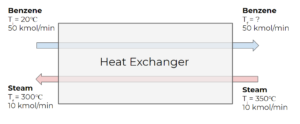The heat capacity of benzene can be represented using the following expression:

$C_{P} = A + BT + CT^{2} + DT^{3}$

where T is in K and $C_{p}$ is given in J/mol K.

 A B C D Benzene -6.211 5.65 x 10-1 -3.141 x 10-4 0

The enthalpy change for steam can be found using data from the steam table:

 300°C, 0.1 MPa 350°C, 0.1 MPa $\hat{H}$ (kJ/kg) 3074.5 3175.8

The benzene’s boiling point temperature is 80.1°C at 1 atm, and its heat of vaporization is 30.765 kJ/mol.

Does the benzene vapourize in the heat exchanger? What is the final temperature of the benzene exiting the heat exchanger? Assume there is no energy loss due to heat transfer.

### Solution

Because there are no other forms of energy transfer other than the heat transfer between the streams, the energy balance simplifies to:

$\Delta\dot{H}_{water}+\Delta\dot{H}_{acetic \;acid}=0$

The enthalpy change for steam  $\Delta\dot{H}$ in the energy balance can be expanded to:

$\Delta\dot{H}_{water}=\dot{H}_f-\dot{H}_i$

\begin{align*}
\Delta\dot{H}_{benzene}&=\int_{T_i}^{T_f}C_pdT\\
&=\int_{T_i}^{T_f}A+BT+CT^2+DT^3 dT\\
&=AT+\frac{1}{2}BT^2+\frac{1}{3}CT^3+\frac{1}{4}DT^4\;\Bigg|_{T_i}^{T_f}\\
&= A(T_f-T_i)+\frac{1}{2}B(T_f-T_i)^2+\frac{1}{3}C(T_f-T_i)^3+\frac{1}{4}D(T_f-T_i)^4
\end{align*}

Step 1: Input given information that will be used in the calculation. This includes the given data to calculate enthalpy change, the molar flow rate of both substances, molecular weights, and the initial temperature of the benzene stream.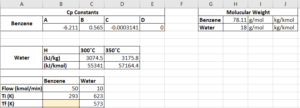Step 2: Calculate the molar enthalpy change. For water, this is calculated by subtracting the molar enthalpy values calculated by subtracting the initial enthalpy from the final. We also convert the units by multiplying the water’s mass enthalpies by the molecular weights at the final and initial temperatures to keep it consistant with the benzene stream.
For benzene, we need to provide a guess of the final temperature to substitute into the equation. For example, we can guess 400 K.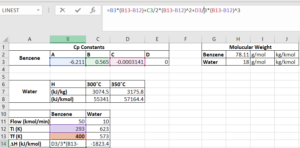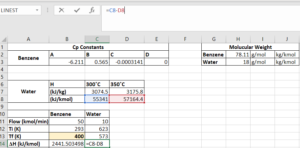Step 3: Calculate the total enthalpy change for both substances by multiplying the molar flow rate by the molar enthalpy change.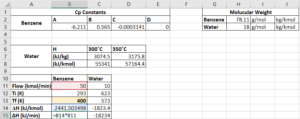Step 4: Use a cell to represent the sum of enthalpy change. If we have guessed the correct final temperature, the value of this cell should be 0. We will use Solver to let excel perform the guess-and-check process for us.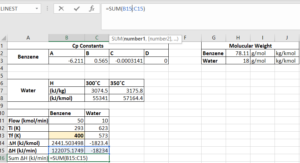Step 5: Select “Solver” from the “Data” menu in the header, and indicate that we want to solve for the final temperature of benzene that makes our $\text{sum of}\; \Delta H=0$:

• Set objective to be the cell containing $\text{sum of}\; \Delta H$
• To: value of 0
• By changing variable cells containing the final temperature of benzene
• Click “Solve”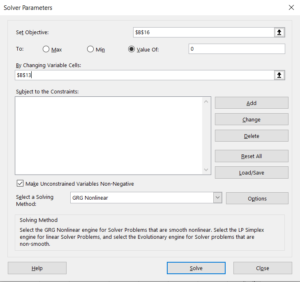Step 6: Click “OK” if Solver finds a solution. If the solver cannot find a solution, it is maybe because our initial guess is too far away from the actual final temperature, if so, we can change the temperature and try to solve it again.

Step 7: After the window closes, the value of the final temperature of benzene and all cells affected by that temperature are changed to the corresponding values calculated with the actual final temperature.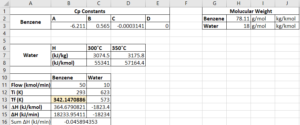Therefore, the final temperature of the benzene stream is $342.1K$, which is equal to $69.1°C$. This temperature is lower than the boiling temperature of benzene at 1 atm, so the stream will not start to vapourize.

Exercise: Heat of Combustion of Ethylene

Consider the combustion of ethylene:

C2H4 (g) + 3 O2 (g) → 2 CO2 (g) + 2 H2O (g)

The enthalpy of combustion can be determined using the enthalpies of the following reactions:

• Reaction 1: C2H2 + H2 → C2H$\Delta H_{r1} = -174.19 kJ$
• Reaction 2: 2 C2H2 + 5 O2 → 4 CO2 + 2 H2O $\Delta H_{r2} = -2511 kJ$
• Reaction 3: 2 CO2 + H2 → 2 O2 + C2H2 $\Delta H_{r3} = 1013.7 kJ$

Determine the enthalpy of the combustion of ethylene from reactions 1, 2, and 3 using Hess’s Law at standard temperature and pressure.

### Solution

Step 1: Determine the combination of reactions that will give us the desired combustion reaction.

We know that we need C2H4 in the reactants, so we multiply reaction 1 by -1. We notice that by adding the other 2 reactions to reaction 1 (multiplied by -1), we get our desired reaction: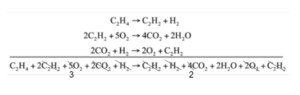Step 2: Calculate the heat of combustion from the reaction enthalpies.

$\Delta H^{\circ} =-1*\Delta H^{\circ}_{r1} + \Delta H^{\circ}_{r2} + \Delta H^{\circ}_{r3}$

$\Delta H^{\circ} = -1* (-174.19 kJ) + (-2511 kJ) + 1013.7 kJ$

$\Delta H^{\circ} = -1323.11 kJ$

Exercise: Enthalpy of Formation of Ethylene Combustion

Consider the combustion of ethylene:

C2H4 (g) + 3 O2 (g) → 2 CO2 (g) + 2 H2O (g)

Using the enthalpies of formation listed below, determine the enthalpy of combustion for ethylene at standard temperature and pressure. Compare the enthalpy of combustion to the one found in the previous exercise found using Hess’s Law.

 Compound $\Delta H_{f}^{\circ}$ (kJ/mol) C2H2(g) 226.7 C2H4(g) 52.51 O2(g) 0 CO2(g) -393.5 H2O(g) -241.8 H2(g) 0

### Solution

Step 1: Calculate the enthalpy of reaction for each of the reactions using the enthalpy of formation of the reactants and products.

$\Delta H^{\circ}_{r} = \Sigma_{i}\nu_{i}\Delta\hat{H}^{\circ}_{f,i} = \Sigma_{products}|\nu_{i}|\Delta\hat{H}^{\circ}_{f,i}-\Sigma_{reactants}|\nu_{i}|\Delta\hat{H}^{\circ}_{f,i}$

$\Delta H^{\circ}_{r} = 2 mol (-393.5 \frac{kJ}{mol}) + 2 mol (-241.8 \frac{kJ}{mol}) - 1 mol (52.51 \frac{kJ}{mol} - 3 mol (0 \frac{kJ}{mol}) = -1323.11 kJ$

Step 2Compare the calculated enthalpy of combustion using heats of formation to the enthalpy of combusion using Hess’s Law from the previous exercise.

They are both equal to -1323.11 kJ!